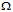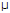# Digital Electronics - Digital Signal Processing

### Exercise :: Digital Signal Processing - General Questions

6.

The ADC0804 is an example of a ________.

 A. single-slope analog-to-digital converter B. dual-slope analog-to-digital converter C. digital-ramp analog-to-digital converter D. successive-approximation analog-to-digital converter

Answer: Option D

Explanation:

No answer description available for this question. Let us discuss.

7.

In a digital representation of voltages using an 8-bit binary code, how many values can be defined?

 A. 16 B. 64 C. 128 D. 256

Answer: Option D

Explanation:

No answer description available for this question. Let us discuss.

8.

A 4-bit R/2R ladder digital-to-analog converter uses ________.

 A. one resistor value B. two resistor values C. three resistor values D. four resistor values

Answer: Option B

Explanation:

No answer description available for this question. Let us discuss.

9.

A binary-weighted-input digital-to-analog converter has a feedback resistor, Rf, of 12 k. If 50A of current is through the resistor, voltage out of the circuit is ________.

 A. 0.6 V B. –0.6 V C. 0.1 V D. –0.1 V

Answer: Option B

Explanation:

No answer description available for this question. Let us discuss.

10.

The resolution of a 6-bit DAC is ________.

 A. 63% B. 64% C. 15.9% D. 1.59%

Answer: Option D

Explanation:

No answer description available for this question. Let us discuss.

#### Current Affairs 2021

Interview Questions and Answers